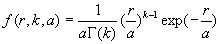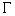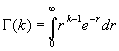`Appendix A`

The
gamma distribution function:In which k is the shape parameter, a is the scale parameter,is the gamma function which has the formula: